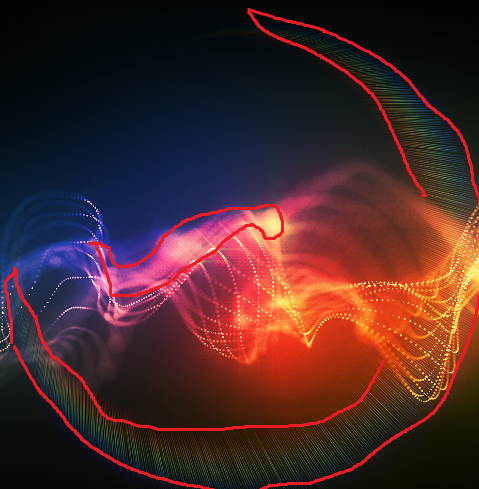#### Howdy, Stranger!

We are about to switch to a new forum software. Until then we have removed the registration on this forum.

# Create new image with pixels

Hello guys,

I'm trying to build a new image, capturing only part that interests me from another, I used this base to begin with: https://processing.org/tutorials/pixels/

I am in doubt as to the image below, the part where it seems "rainbow", would I use the function (hue), or (r, g, b) individually to capture?

And what about the circled part (very badly done, sorry) more in the middle, would have to use the function (brightness), since it is kind of "brightness" in the image?

Thanks any hint, below is the sample code, and the image as template.

Thank you,

``````    PImage source;
PImage destination;
float threshold = 0;

void setup() {
size(400, 300);
source.resize(400, 300);
destination = createImage(source.width, source.height, RGB);
}

void draw() {
//threshold = 127;
threshold = 70;

for (int x = 0; x < source.width; x++) {
for (int y = 0; y < source.height; y++ ) {
int loc = x + y*source.width;
if (brightness(source.pixels[loc]) > threshold) {
//if (hue(source.pixels[loc]) > threshold) {
//destination.pixels[loc]  = source.pixels[loc];  // White
destination.pixels[loc]  = source.pixels[loc];
}
}
}

destination.updatePixels();
image(destination,0,0);
//image(source,0,0);
}

void keyPressed(){
if (key == 'A' || key == 'a')
threshold = threshold + 10;

if (key == 'D' || key == 'd')
threshold = threshold - 10;

println(threshold);
}
``````Tagged:

• Here is a suggestion: If you want to select certain color, you can use distance:

``````final color REF=color(150,50,0);  //Reference color
color pix=get(x,y); //x and y can be any pix

float colorThreshold =50;  //How far your pic color can be from the reference color

if(dist(red(REF),green(REF),blue(REF),red(pix),green(pix),blue(pix))<colorThreshold){
//If true, then colors are alike...
}
``````

In your case, I think you will need a mix of brightness and hue (?) If you use HSB, notice you could do something like this:

``````if(abs(hue(pix),hue(REF))<colorThresh){
... pix is close to my reference hue
}
``````

However, your case is very specific and you should give it a try and see how the algorithm performs. It is different to use HSB to RGB or maybe you could use both.

Kf

• Hello kfrajer, thanks for the feedback ...

I'm going to do tests, thanks for the attention,

Thanks!

• If this something you want to do once or you are looking to automate it?

Kf

• Hello, thanks again ...

This is just an example, but if I can do this, it would be a big step, so I can think of how to automate for other images, I'm actually realizing that it's something very complex ...

As I mentioned, I need to "filter" only certain colors and brightness in the image, and I was thinking how to mount this range of values, and then compare with the pixels of the image, to see if it is in the range, and thus compose a new image.

I still have not figured out how to do this, I thought of putting together a two-dimensional array with the desired ranges of values, and if pixel value is in this range, I use, if not discard ...

The problem I could not "see" yet, how would I compare the range of values ...

This image passes a close idea of what I need to do, if I can compare and separate only part of the "rainbow", it would be a big step.

Thanks for the interest ...

Sorry for English, I'm writing with Google Translate.

Thank you very much

• Sorry, I made a mistake, I sent it twice ...

• Some ideas:

1. in order to filter your image, use an "else" to black out the things you aren't copying (or just recreate the image every time).
2. combine checking hue with checking saturation, otherwise results will be extremely misleading -- shades of gray will appear in color filters
3. if using hue, use an HSB colorMode, and be careful about checking values that wrap around when computing color distance -- otherwise 5 (red) won't be considered close to 250 (red)

``````PImage source, destination;
float threshold;
int mode;

void setup() {
size(400, 300);
source.resize(400, 300);
destination = createImage(source.width, source.height, RGB);
colorMode(HSB, 255);
threshold = 170;
}

void draw() {

for (int x = 0; x < source.width; x++) {
for (int y = 0; y < source.height; y++ ) {
int loc = x + y*source.width;
if(mode==0 && brightness(source.pixels[loc]) > threshold){
destination.pixels[loc]  = source.pixels[loc];
} else if(mode==1 && hueClose((hue(source.pixels[loc])), threshold, 255, 20) && saturation(source.pixels[loc]) > 32){
destination.pixels[loc]  = source.pixels[loc];
} else {
destination.pixels[loc] = color(0,0,0);
}
}
}

destination.updatePixels();
image(destination,0,0);
}

void keyPressed(){
if (key == 'A' || key == 'a')
threshold = threshold + 10;

if (key == 'D' || key == 'd')
threshold = threshold - 10;

if (key == 'B' || key == 'b')
mode = 0;

if (key == 'H' || key == 'h')
mode = 1;

println(threshold);
}

boolean hueClose(float a, float b, float max, float close){
if(hueDist(a,b,max)<close){
return true;
} else {
return false;
}
}

float hueDist(float a, float b, float max){
return ((a - b) + (max/2))%max - (max/2);
}
``````
• Hello jeremydouglass,

Thank you very much for the tips, this will certainly help to adapt to my needs.

I'll work on it better, and as soon as I get something new, I'm here ...

Thanks guys, I really appreciate the help I always have here!

Thanks!